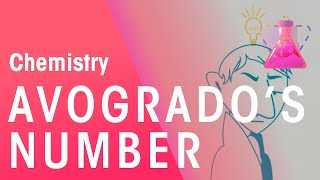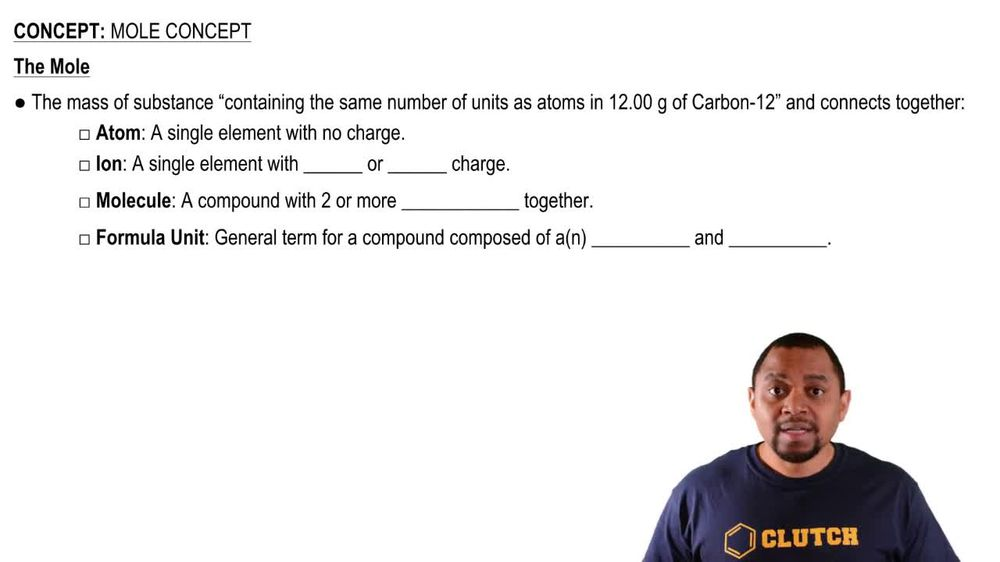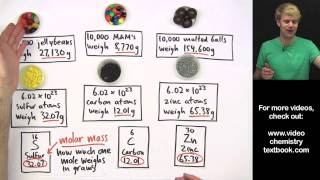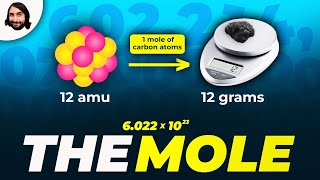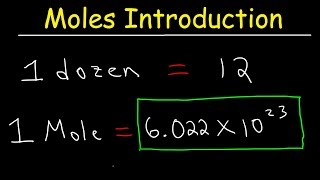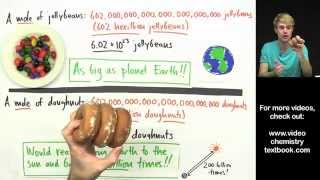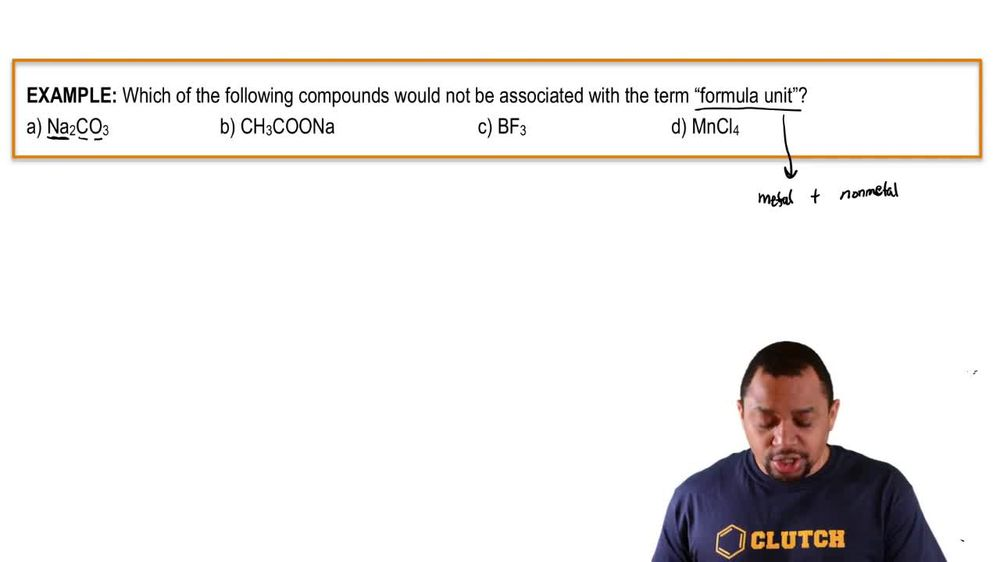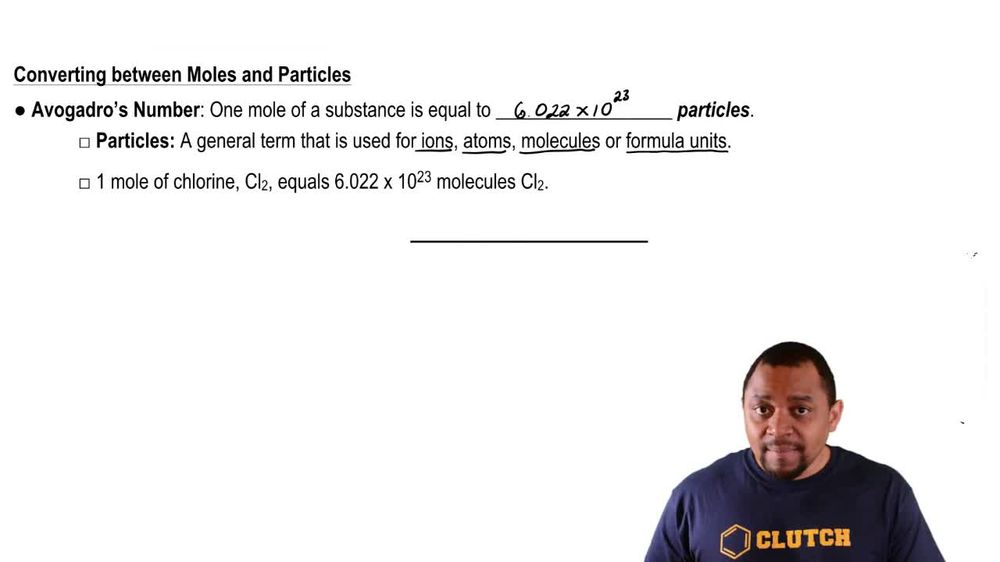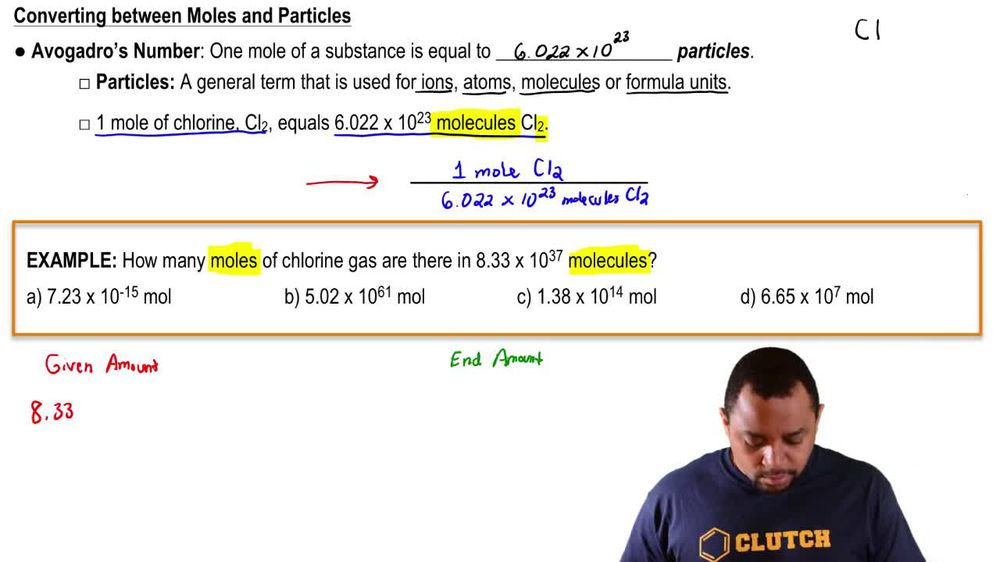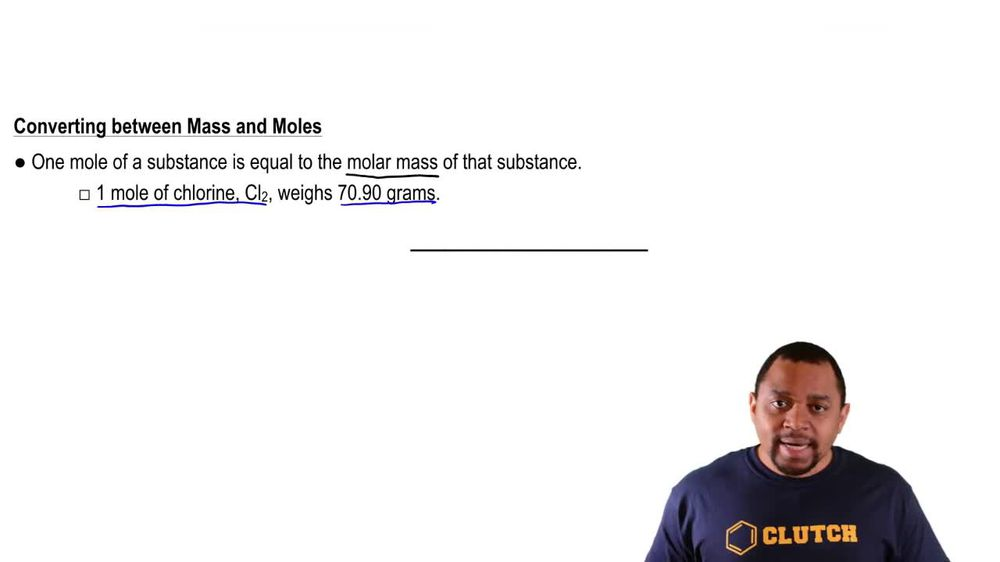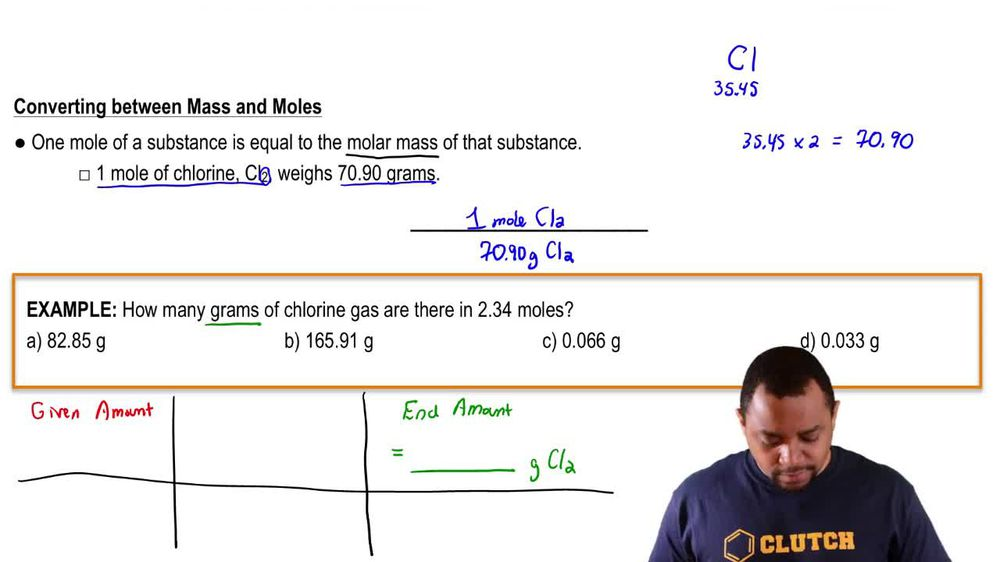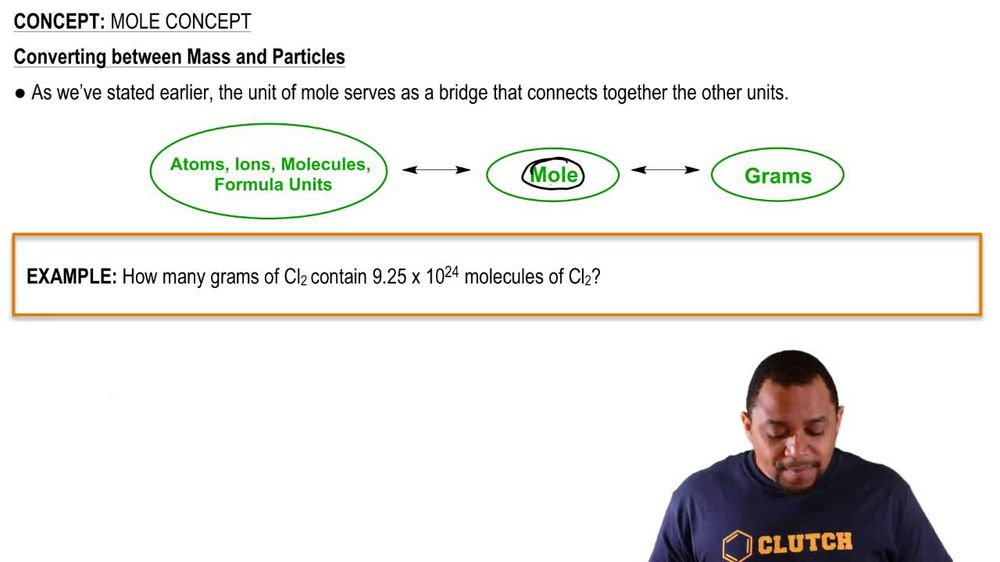Start typing, then use the up and down arrows to select an option from the list.
1. 2. Atoms & Elements2. Mole Concept
Problem

# (c) What is the mass, in grams, of 7.70 * 1020 molecules of caffeine, C8H10N4O2?

Relevant Solution1m
Play a video:
Hey everyone where us to calculate the mass of a sample containing 8.45 times senses 23rd molecules of Melatonin first. Let's go ahead and write out our pathway. We were given molecules of melatonin Now in order to get two g of melatonin will first need to get two moles of Melatonin. Next we can finally get two g of melatonin. Now let's go ahead and answer this question. Starting off with 8.45 times 10 to the 23rd molecules of melatonin to get two moles of melatonin, we have to use our dimensional analysis and avocados number so we know that we have 6.22 times 10 to 23rd molecules of melatonin Per one Mole of Melatonin. And again this is avocados number. Next we need to know melatonin molar mass and when we calculate that out, we end up with 232.31 g of melatonin per one mole of melatonin. And when we calculate this out and cancel out all of our units, we end up with a total of 3.26 times 10 to the second grams of melatonin which is going to be our final answer. So I hope this made sense. And let us know if you have any questions